# Methods and formulas for the distributions in Tolerance Intervals (Nonnormal Distribution)

Select the method or formula of your choice.

## Maximum likelihood estimates

Maximum likelihood estimates of the parameters in the distribution are calculated by maximizing the likelihood function with respect to the parameters. For a given data set, the maximum likelihood estimates are the most likely values for the distribution parameters.

The Newton-Raphson algorithm is used to calculate maximum likelihood estimates of the distribution parameters. The Newton-Raphson algorithm is an iterative numerical method for calculating the maximum of a function. 1

###### Note

Minitab calculates the parameter estimates using the maximum likelihood method for all the distributions except the lognormal distribution. For the lognormal distribution, Minitab calculates unbiased parameter estimates.

## Probability distributions

### Lognormal distribution

 PDF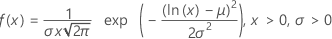CDF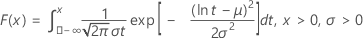Mean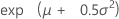Stdev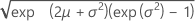TermDescription
μScale parameter
σShape parameter

### Gamma distribution

 PDF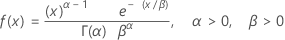CDF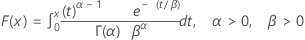Mean αβ Stdev αβ2
TermDescription
αShape parameter
βScale parameter

### Exponential distribution

 PDF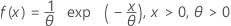CDF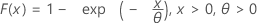Mean θ Stdev θ
TermDescription
θScale parameter

### Smallest extreme value distribution

 PDF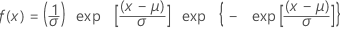CDF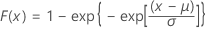Mean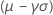Stdev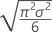TermDescription
μLocation parameter
σScale parameter
γEuler's constant (approximately equals 0.5772)

### Weibull distribution

 PDF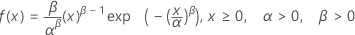CDF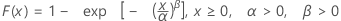Mean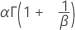Stdev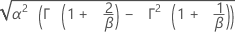TermDescription
αScale parameter
βShape parameter

### Largest extreme value distribution

 PDF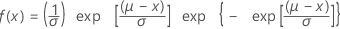CDF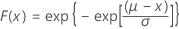Mean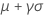StdevTermDescription
μLocation parameter
σScale parameter
γEuler's constant (approximately equals 0.5772)

### Logistic distribution

 PDF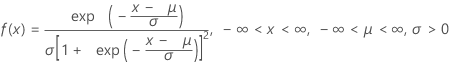CDF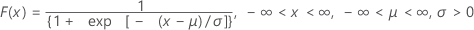Mean μ Stdev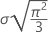TermDescription
μLocation parameter
σScale parameter

### Loglogistic distribution

 PDF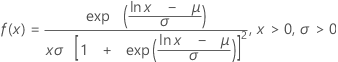CDF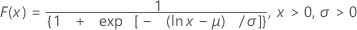Mean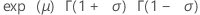Stdev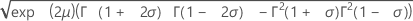TermDescription
μLocation parameter
σScale parameter
1 W. Murray, Ed. (1972). Numerical Methods for Unconstrained Optimization. Academic Press.
By using this site you agree to the use of cookies for analytics and personalized content.  Read our policy